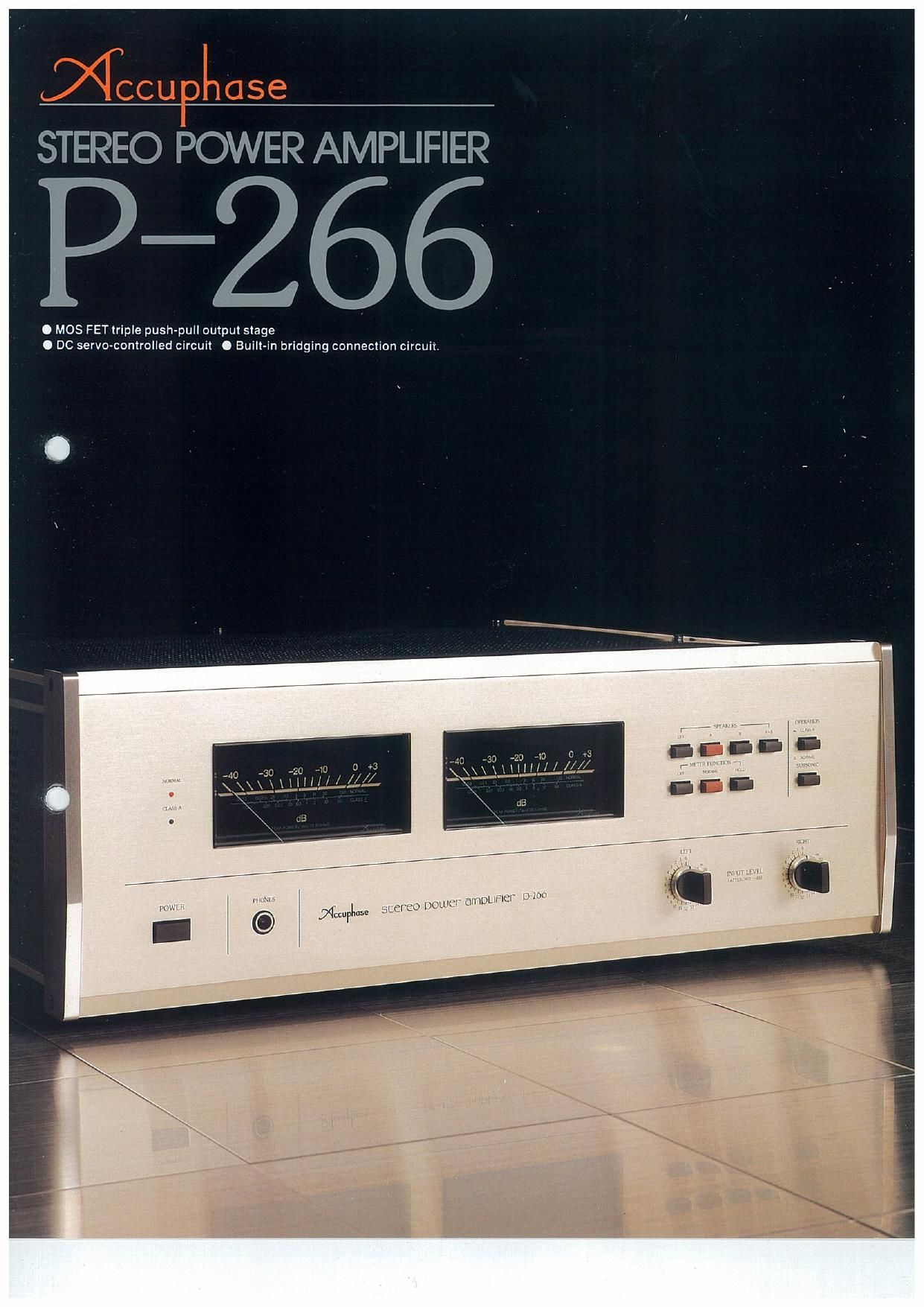# Accuphase P 266 Brochure

This is the 6 pages manual for Accuphase P 266 Brochure.

Page: 1 / 6## Extracted text from Accuphase P 266 Brochure (Ocr-read)

### Page 6

A
B
C
D
E

Frequency characterising

Total harmonic distortion vs. power output
(For normal operation] stereo)

Total harmonic distortion vs. power output
(For normal operation/bridging connection)
Total harmonic distortion vs. power output
(Class-A operation/stereo)

Total harmonic distortion vs. power output
(Class-A operation/brieﬁng connection)

m a w "I azimuth mm

. a .. u x as

The above data shows the spectrums of inter-
modulation distortion for the P-266 as measured by
the new IHF measurement method. Amplitudes of a
19kHz and 20kHz input signals are shown at the
right side. Any inten'nodulation created by these two
signals would appear as spectrum peaks at tkHz
intervals. the frequency difference between the two
signals. across the frequency bandwidth. This data
shows them to be hardly noticeable. confirming that
IM distortion is less than —93 dB (0.002256).

Another form of IM distortion would appear at
39kHz. the sum oi the two input signal frequencies
(19+20=39kHz). Such a distortion. even it it existed.
would be inconsequential because it is far beyond
the audible range. In the P-266. this form of M
distortion is also less than 93dB.

H- r.r.r¢. mm:
m. .murnmemm
m. ileum/rant».
Iva—Inn in

I'm. mm

The above data shows the spectrum character-
istics of transient lntermodulatlon distortion ior the
P-266 when two mixed input signals, a 3.1BkHz
square wave and a 15kHz sine wave. are used.
Since harmonics ol square waves appear almost
infinitely at odd—number multiples. for example In
this case at 9.54kHz (3rd harmonic) 15.9kHz (5th
han-nonlc). they can create, together with the 15kHz
input sine wave. lntermodulated spectrums at fre-
quencies where input signals are absent For exam-
ple. if the third harmonic of the 3.18kHz square wave
(9.54kHz) and the 15kHz Input signal intermodulate,
a speotnrrn can appear at the diiference oi their
frequencies or 5.46kHz (15—9.54=5,46kHz)t
However. the above data shows no spectrum shop
—93dB at that frequency which confirms that TI
distortion ls less than 0.002296.

mﬂCCUO ase

ACCUF’HASE LABORATORY INC.

K845Y

851-0041-00 (G3) PRINTED IN JAPAN